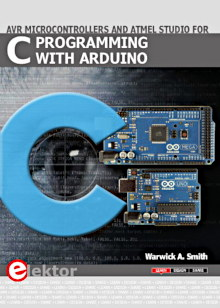Starting Electronics needs your help! Please make a donation to help cover our hosting and other costs. Click the donate button to send a donation of any amount.# Flashing an LED using the Raspberry PI and C Programming Language

Created on: 28 April 2014

An LED connected to one of the Raspberry PI's GPIO pins can be flashed on and off using a program written in the C programming language.

In this article, an LED on the Raspberry PI serial port and breakout board is used, but any LED connected to a GPIO pin on the Raspberry PI will work.

This video shows the LED being flashed by the Raspberry PI:

Can't see the video? View on YouTube →

## C Source Code

The C source code for flashing the LED is listed below:

flash.c

```// Code adapted from example by Gert van Loo & Dom
// Example code from: http://elinux.org/Rpi_Low-level_peripherals#C_2

// flashes (blinks) LED on Raspberry PI GPIO7

#define BCM2708_PERI_BASE        0x20000000
#define GPIO_BASE                (BCM2708_PERI_BASE + 0x200000) /* GPIO controller */

#include <stdio.h>
#include <stdlib.h>
#include <fcntl.h>
#include <sys/mman.h>
#include <unistd.h>

#define PAGE_SIZE (4*1024)
#define BLOCK_SIZE (4*1024)

int  mem_fd;
void *gpio_map;

// I/O access
volatile unsigned *gpio;

// GPIO setup macros. Always use INP_GPIO(x) before using OUT_GPIO(x) or SET_GPIO_ALT(x,y)
#define INP_GPIO(g) *(gpio+((g)/10)) &= ~(7<<(((g)%10)*3))
#define OUT_GPIO(g) *(gpio+((g)/10)) |=  (1<<(((g)%10)*3))
#define SET_GPIO_ALT(g,a) *(gpio+(((g)/10))) |= (((a)<=3?(a)+4:(a)==4?3:2)<<(((g)%10)*3))

#define GPIO_SET *(gpio+7)  // sets   bits which are 1 ignores bits which are 0
#define GPIO_CLR *(gpio+10) // clears bits which are 1 ignores bits which are 0

void setup_io();

int main(int argc, char **argv)
{
int g,rep;

// Set up gpi pointer for direct register access
setup_io();

// set GPIO pin 7 as output
INP_GPIO(7); // must use INP_GPIO before we can use OUT_GPIO
OUT_GPIO(7);

// flash LED on and off 10 times
for (rep = 0; rep < 10; rep++) {
GPIO_SET = (1 << 7);
sleep(1);
GPIO_CLR = (1 << 7);
sleep(1);
}

return 0;

} // main

//
// Set up a memory regions to access GPIO
//
void setup_io()
{
/* open /dev/mem */
if ((mem_fd = open("/dev/mem", O_RDWR|O_SYNC) ) < 0) {
printf("can't open /dev/mem \n");
exit(-1);
}

/* mmap GPIO */
gpio_map = mmap(
NULL,                 //Any adddress in our space will do
BLOCK_SIZE,           //Map length
MAP_SHARED,           //Shared with other processes
mem_fd,               //File to map
GPIO_BASE             //Offset to GPIO peripheral
);

close(mem_fd); //No need to keep mem_fd open after mmap

if (gpio_map == MAP_FAILED) {
printf("mmap error %d\n", (int)gpio_map); //errno also set!
exit(-1);
}

// Always use volatile pointer!
gpio = (volatile unsigned *)gpio_map;
} // setup_io()
```

Books that may interest you:## Compiling and Running the Code

Copy and paste the above code to a text file and save it on the Raspberry PI, e.g. save it as flash.c.

To compile the code using the GCC C compiler, enter the following at the command prompt when in the same directory as the flash.c file:

```gcc flash.c -o flash
```

Once the file has been compiled, there will be an executable file called flash in the current directory. To run the file, enter:

```sudo ./flash
```

Press Ctrl + C to exit the program.

Raspberry PI Software